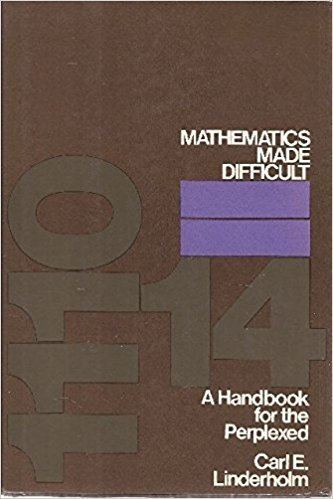Updated on
Covid-19
8.6
1 Ratings
 10 0 9 0 8 1 7 0 6 0 5 0 4 0 3 0 2 0 1 0
Rate This

Rate This

4.3/5 AbeBooks

Publication date  1972
ISBN  978-0-529-04552-2
Originally published  1972
Page count  207
Subjects  Mathematics, Satire

Publisher  World Publishing
Pages  207
Dewey Decimal  510
Author
OCLC  279066Mathematics Made Difficult is a book by Carl E. Linderholm that uses advanced mathematical methods to prove results normally shown using elementary proofs. Although the aim is largely satirical, it also shows the non-trivial mathematics behind operations normally considered obvious, such as numbering, counting, and factoring integers.

As an example, the proof that two is a prime number starts:

It is easily seen that the only numbers between 0 and 2, including 0 but excluding 2, are 0 and 1. Thus the remainder left by any number on division by 2 is either 0 or 1. Hence the quotient ring Z/2Z, where 2Z is the ideal in Z generated by 2, has only the elements  and , where these are the images of 0 and 1 under the canonical quotient map. Since  must be the unit of this ring, every element of this ring except  is a unit, and the ring is a field ...

## References

Similar Topics
Tuesday in November
Probal Rashid
Cristina Comencini
Topics